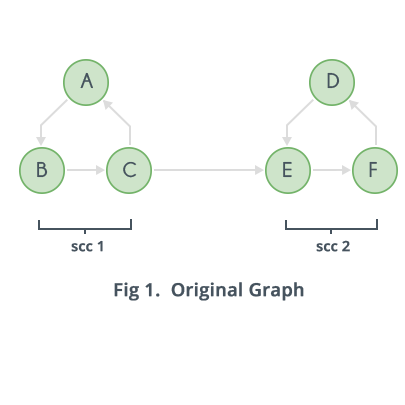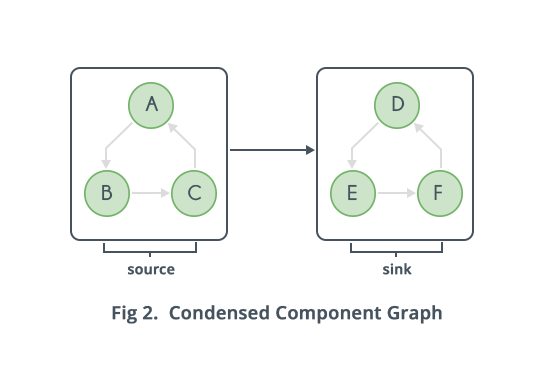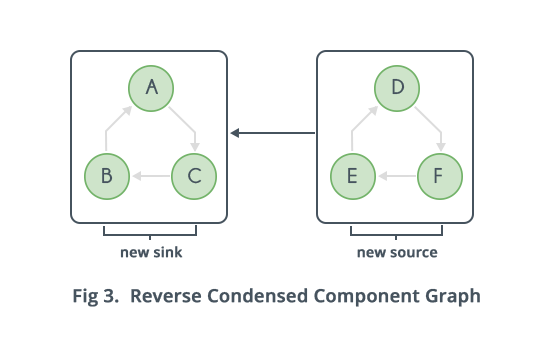Algorithms
Topics:
Strongly Connected Components
• Searching
• Sorting
• Greedy Algorithms
• Graphs
• String Algorithms
• Dynamic Programming

# Strongly Connected Components

Connectivity in an undirected graph means that every vertex can reach every other vertex via any path. If the graph is not connected the graph can be broken down into Connected Components.

Strong Connectivity applies only to directed graphs. A directed graph is strongly connected if there is a directed path from any vertex to every other vertex. This is same as connectivity in an undirected graph, the only difference being strong connectivity applies to directed graphs and there should be directed paths instead of just paths. Similar to connected components, a directed graph can be broken down into Strongly Connected Components.Basic/Brute Force method to find Strongly Connected Components:

Strongly connected components can be found one by one, that is first the strongly connected component including node $1$ is found. Then, if node $2$ is not included in the strongly connected component of node $1$, similar process which will be outlined below can be used for node $2$, else the process moves on to node $3$ and so on.

So, how to find the strongly connected component which includes node $1$? Let there be a list which contains all nodes, these nodes will be deleted one by one once it is sure that the particular node does not belong to the strongly connected component of node $1$. So, initially all nodes from $1$ to $N$ are in the list. Let length of list be $LEN$, current index be $IND$ and the element at current index $ELE$. Now for each of the elements at index $IND+1,...,LEN$, assume the element is $OtherElement$, it can be checked if there is a directed path from $OtherElement$ to $ELE$ by a single $O(V+E)$ $DFS$, and if there is a directed path from $ELE$ to $OtherElement$, again by a single $O(V+E)$ $DFS$. If not, $OtherElement$ can be safely deleted from the list.

After all these steps, the list has the following property: every element can reach $ELE$, and $ELE$ can reach every element via a directed path. But the elements of this list may or may not form a strongly connected component, because it is not confirmed that there is a path from other vertices in the list excluding $ELE$ to the all other vertices of the list excluding $ELE$.

So to do this, a similar process to the above mentioned is done on the next element(at next index $IND+1$) of the list. This process needs to check whether elements at indices $IND+2,...,LEN$ have a directed path to element at index $IND+1$. It should also check if element at index $IND+1$ has a directed path to those vertices. If not, such nodes can be deleted from the list. Now one by one, the process keeps on deleting elements that must not be there in the Strongly Connected Component of $1$.

In the end, list will contain a Strongly Connected Component that includes node $1$. Now, to find the other Strongly Connected Components, a similar process must be applied on the next element(that is $2$), only if it has not already been a part of some previous Strongly Connected Component(here, the Strongly Connected Component of $1$). Else, the process continues to node $3$ and so on.

The time complexity of the above algorithm is $O(V^{3})$.

Kosaraju's Linear time algorithm to find Strongly Connected Components:

This algorithm just does $DFS$ twice, and has a lot better complexity $O(V+E)$, than the brute force approach. First define a Condensed Component Graph as a graph with $\le V$ nodes and $\le E$ edges, in which every node is a Strongly Connected Component and there is an edge from $C$ to $C'$, where $C$ and $C'$ are Strongly Connected Components, if there is an edge from any node of $C$ to any node of $C'$.It can be proved that the Condensed Component Graph will be a Directed Acyclic Graph($DAG$). To prove it, assume the contradictory that is it is not a $DAG$, and there is a cycle. Now observe that on the cycle, every strongly connected component can reach every other strongly connected component via a directed path, which in turn means that every node on the cycle can reach every other node in the cycle, because in a strongly connected component every node can be reached from any other node of the component. So if there is a cycle, the cycle can be replaced with a single node because all the Strongly Connected Components on that cycle will form one Strongly Connected Component.

Therefore, the Condensed Component Graph will be a $DAG$. Now, a $DAG$ has the property that there is at least one node with no incoming edges and at least one node with no outgoing edges. Call the above $2$ nodes as Source and Sink nodes. Now observe that if a $DFS$ is done from any node in the Sink(which is a collection of nodes as it is a Strongly Connected Component), only nodes in the Strongly Connected Component of Sink are visited. Now, removing the sink also results in a $DAG$, with maybe another sink. So the above process can be repeated until all Strongly Connected Component's are discovered. So at each step any node of Sink should be known. This should be done efficiently.

Now a property can be proven for any two nodes $C$ and $C'$ of the Condensed Component Graph that share an edge, that is let $C \rightarrow C'$ be an edge. The property is that the finish time of $DFS$ of some node in $C$ will be always higher than the finish time of all nodes of $C'$.

Proof: There are $2$ cases, when $DFS$ first discovers either a node in $C$ or a node in $C'$.

Case 1: When $DFS$ first discovers a node in $C$: Now at some time during the $DFS$, nodes of $C'$ will start getting discovered(because there is an edge from $C$ to $C'$), then all nodes of $C'$ will be discovered and their $DFS$ will be finished in sometime (Why? Because it is a Strongly Connected Component and will visit everything it can, before it backtracks to the node in $C$, from where the first visited node of $C'$ was called). Therefore for this case, the finish time of some node of $C$ will always be higher than finish time of all nodes of $C'$.

Case 2: When $DFS$ first discovers a node in $C'$: Now, no node of $C$ has been discovered yet. $DFS$ of $C'$ will visit every node of $C'$ and maybe more of other Strongly Connected Component's if there is an edge from $C'$ to that Strongly Connected Component. Observe that now any node of $C$ will never be discovered because there is no edge from $C'$ to $C$. Therefore $DFS$ of every node of $C'$ is already finished and $DFS$ of any node of $C$ has not even started yet. So clearly finish time of some node(in this case all) of $C$, will be higher than the finish time of all nodes of $C'$.

So, if there is an edge from $C$ to $C'$ in the condensed component graph, the finish time of some node of $C$ will be higher than finish time of all nodes of $C'$. In other words, topological sorting(a linear arrangement of nodes in which edges go from left to right) of the condensed component graph can be done, and then some node in the leftmost Strongly Connected Component will have higher finishing time than all nodes in the Strongly Connected Component's to the right in the topological sorting.

Now the only problem left is how to find some node in the sink Strongly Connected Component of the condensed component graph. The condensed component graph can be reversed, then all the sources will become sinks and all the sinks will become sources. Note that the Strongly Connected Component's of the reversed graph will be same as the Strongly Connected Components of the original graph.Now a $DFS$ can be done on the new sinks, which will again lead to finding Strongly Connected Components. And now the order in which $DFS$ on the new sinks needs to be done, is known. The order is that of decreasing finishing times in the $DFS$ of the original graph. This is because it was already proved that an edge from $C$ to $C'$ in the original condensed component graph means that finish time of some node of $C$ is always higher than finish time of all nodes of $C'$. So when the graph is reversed, sink will be that Strongly Connected Component in which there is a node with the highest finishing time. Since edges are reversed, $DFS$ from the node with highest finishing time, will visit only its own Strongly Connected Component.

Now a $DFS$ can be done from the next valid node(valid means which is not visited yet, in previous $DFSs$) which has the next highest finishing time. In this way all Strongly Connected Component's will be found. The complexity of the above algorithm is $O(V+E)$, and it only requires $2 DFSs$.

The algorithm in steps can be described as below:

$1)$ Do a $DFS$ on the original graph, keeping track of the finish times of each node. This can be done with a stack, when some $DFS$ finishes put the source vertex on the stack. This way node with highest finishing time will be on top of the stack.

stack STACK
void DFS(int source) {
visited[s]=true
for all neighbours X of source that are not visited:
DFS(X)
STACK.push(source)
}


$2)$ Reverse the original graph, it can be done efficiently if data structure used to store the graph is an adjacency list.

CLEAR ADJACENCY_LIST
for all edges e:
first = one end point of e
second = other end point of e


$3)$ Do $DFS$ on the reversed graph, with the source vertex as the vertex on top of the stack. When $DFS$ finishes, all nodes visited will form one Strongly Connected Component. If any more nodes remain unvisited, this means there are more Strongly Connected Component's, so pop vertices from top of the stack until a valid unvisited node is found. This will have the highest finishing time of all currently unvisited nodes. This step is repeated until all nodes are visited.

while STACK is not empty:
source = STACK.top()
STACK.pop()
if source is visited :
continue
else :
DFS(source)

Contributed by: Rishi Vikram